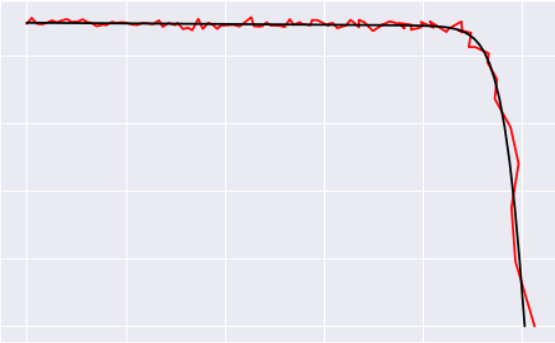# Conv_learner from DataFrame

Hello, I am trying to create a NN to "denoise"vectors.
My input are 400 long vectors and my output is of the same length.
I have a lot of samples, around 1kk, from a machine that samples a physical phenomenon, but the accuracy is not that good, and the points have noise. I would like to remove the noise, and obtain a smooth curve.

My first try, was use the equation that describes the phenomenon (very complex non-linear equation), and create synthetic data, to train a model to denoise the curves. I tried random forest and it works good, then I tried fastai with Columnar data, (modified Rossmann ipynb):

`````` md = ColumnarModelData.from_data_frame(PATH, val_idx, noise_df, clean_df.values, cat_flds=[], bs=256,
is_reg=True, is_multi=False)
``````

It also worked good,
But I think that my problem is closer to and image regression, maybe a ConvLearner with a custom head as in BBOX problem?
How do I feed a DataFrame to the ConvLearner?

This is the type of “curve denoise” I am working with.Sincerely,
Thomas